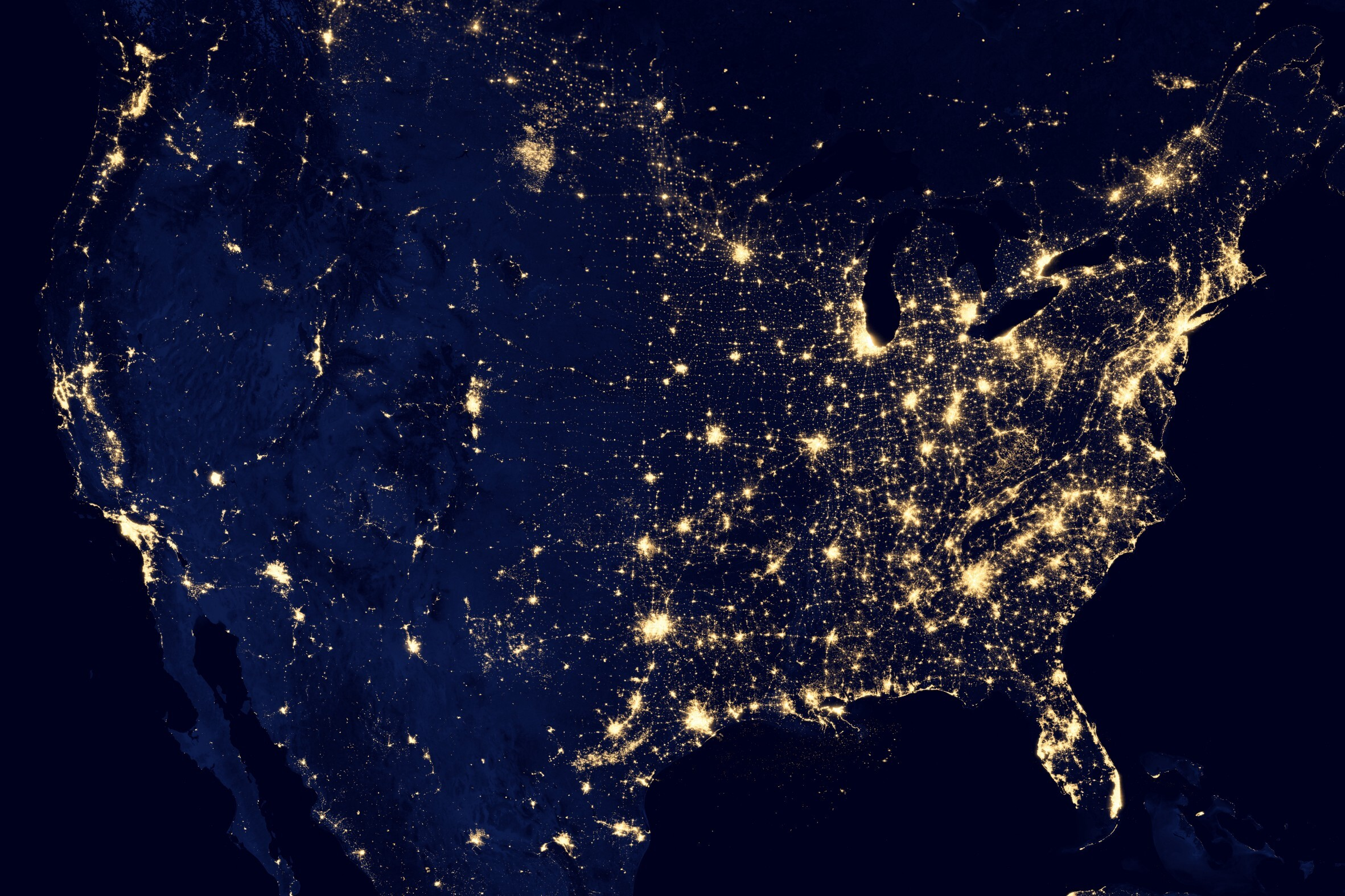Tutorial
< Back to the blog

## FASTER MACHINE LEARNING USING HUB BY ACTIVELOOP: CODE WALKTHROUGH

### Introduction

Machine learning engineers work less on machine learning and more on data preparation. In fact, a typical ML engineer spends more than 50% of their time preprocessing the data, rather than analyzing it. Preparing data pipelines so that the team of engineers can utilise the data takes a lot of time, which makes running machine learning experiments and collaborating in a team quite a difficult task.

My team of machine learning engineers and I recently faced similar problems while working on a project with Omdena, as well as the World Resources Institute. Thankfully, we had the support of the Activeloop team and their open-source package Hub, which made it easier for our group of collaborators to work simultaneously on running experiments and reach our end goal much faster. You can think of Activeloop’s Hub as the Docker Hub for datasets.### Problem Statement

In the 2 month project, the problem statement was to model economic well being using satellite imagery and ground truth survey data.

This basically means that we had to use multi-band satellite images of a particular area and train a model which would be able to learn features relating to urbanization and changing agriculture and it would provide a proxy or an estimate of what the economic conditions are of that area.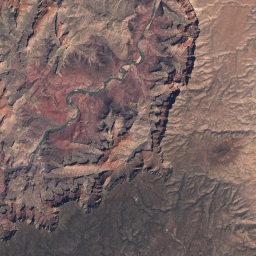This would not only save a lot of time and money, but also diminish the noise in the data that is otherwise collected through surveys. This project falls under the UN's Sustainable Development Goal 8.

This would not only save a lot of time and money, but also diminish the noise in the data that is otherwise collected through surveys. This project falls under the UN's Sustainable Development Goal 8

### Data preprocessing and data storage - the real challenge

To solve this problem, one of the solutions that we came up with was to collect wealth data from the DHS Programme for Indian districts and then use the district level satellite imagery to correlate them.We would create an image classification problem, where the inputs would be the satellite image of districts and the output would be the asset wealth index. The constraint was to only use open source data, so we decided to use Google Earth Engine for downloading images from the satellite Landsat 8.

Then came the actual challenge that we were facing, data storage issues.

Satellite images, unlike the images that we store in our devices, are “multi-banded”. They do not just contain the RGB bands but may contain up to a total of 12 bands - some of them being near infrared bands, short wave infrared bands, etc. This results in very large images, where a single district level image containing 12 bands can take upto 150 MBs. This makes storing these images and storing them in such a way that every collaborator working on this task could access it and run experiments on it, a really difficult task.

There are some methods that exist to tackle this issue:

• Google Drive can be used to store the data and utilise google collaboratory for all the modelling experiments. However when it comes to satellite images, often the space available on a standard Google Drive account is not enough.
• Cloud Facilities are a good, however expensive option. You can actually check how much money you might be losing using such facilities using this tool created by Activeloop.

We wanted a method where we could easily store the data, so that everyone could use it with minimal effort and minimal costs.

Here comes Activeloop’s open-source package hub to the rescue!

### Easily storing unstructured data with hub

The python package hub can be used to store unstructured data (in this case - aerial images) onto Activeloop’s platform, it can be easily loaded by anyone anywhere using literally a single line of code, all we need to do is mention the registered account name and the name of the dataset,

``dataset.load("arpan/district-awi-2015-16-rgb-custom")``

As simple as that! It then allows us to preprocess images as if they were stored on our own devices. But to reach this step, we need to first set up our account and upload the dataset. Let’s see how that can be done.

For setting up hub on your system just run the following commands:

``````pip install hub==0.11.0
hub register

After this, you can login using your credentials. Please note here that at the time I was using hub version 0.11.0,however now the beta version, 1.0.0b1 is available with a much better interface for data pipelines and in-depth support of storing datasets. The package now is the fastest way to access and manage datasets for PyTorch and TensorFlow.

I will now go over the code that I used to upload the data using hub. For uploading the satellite images, first they must be downloaded from Google Earth engine, we chose GEE as we had to use openly available satellite data. I downloaded 20km x 20km raster images for every district.

A generator class is created for uploading the images using hub. It contains 2 main methods, the meta and the forward method. For any file that we want to upload we need to mention what sort of data it contains. This is handled by the meta method. The meta method contains the description of each file in the form of a dictionary. In our case this is how the meta function looks like.

``````from hub import Transform, dataset
class AwiGenerator(Transform):
def  meta(self):

return {
'rgb-image': {"shape": (1,), "dtype": "object", 'dtag': 'image'},
'custom-image': {"shape": (1,), "dtype": "object", 'dtag': 'image'},
'awi': {'shape': (1,), 'dtype': 'float', 'dtag': 'text'},
'target': {'shape': (1, ), 'dtype': 'float', 'dtag': 'text'},
'name': {'shape': (1, ), 'dtype': 'object', 'dtag': 'text'}
}``````

The description dictionary contains 3 things:

1. shape - this is the shape of 1 file of this particular type. For example ‘rgb-image’ refers to the image created using the Red, Green and Blue Bands, the shape for every image will be 1 x height x width x 3 (in the form of an array or tensor). We can just use numpy’s convention and mention (1,) as the shape.

2. dtype - This is the datatype of the file. Images and names (district name) are stored as objects and awi (asset wealth index) and target as floats.

3. dtag - This needs to be mentioned when we want to use the visualizer app provided by activeloop. Once the images have been uploaded we can actually see all the images along with the labels that we provided (target, name etc). We’ll see how our uploads looked like later in this article.

Before we go onto the forward method, we need to understand a little about what exactly the model architecture was. The satellite images are multi-banded, Landsat 8, our satellite of interest contains 12 banded images. More information can be found on Google Earth Engine’s data catalog. For our model we require the Red, Green, Blue, Near Infrared and the Short Wave Infrared Bands. Using the NIR and the SWIR bands we can calculate vegetation (NDVI), built up (NDBI) and water indices (NDWI) from the rasters. These indices highlight different geographical attributes of the region for which we have the image. For example, the NDVI highlights all the vegetation areas in the image by increasing the pixel values of the green colours. Similarly NDWI highlights waterbodies by highlighting blue and NDBI!, highlights built up areas (buildings, roads etc.) by highlighting white. These 3 indices are nothing but single band images. We then combine these 3 single band images into one 3 band image so that the model can learn from all three of these indices. The RGB as well as the Custom Band image is the input to the model. Finally this is the architecture that we get: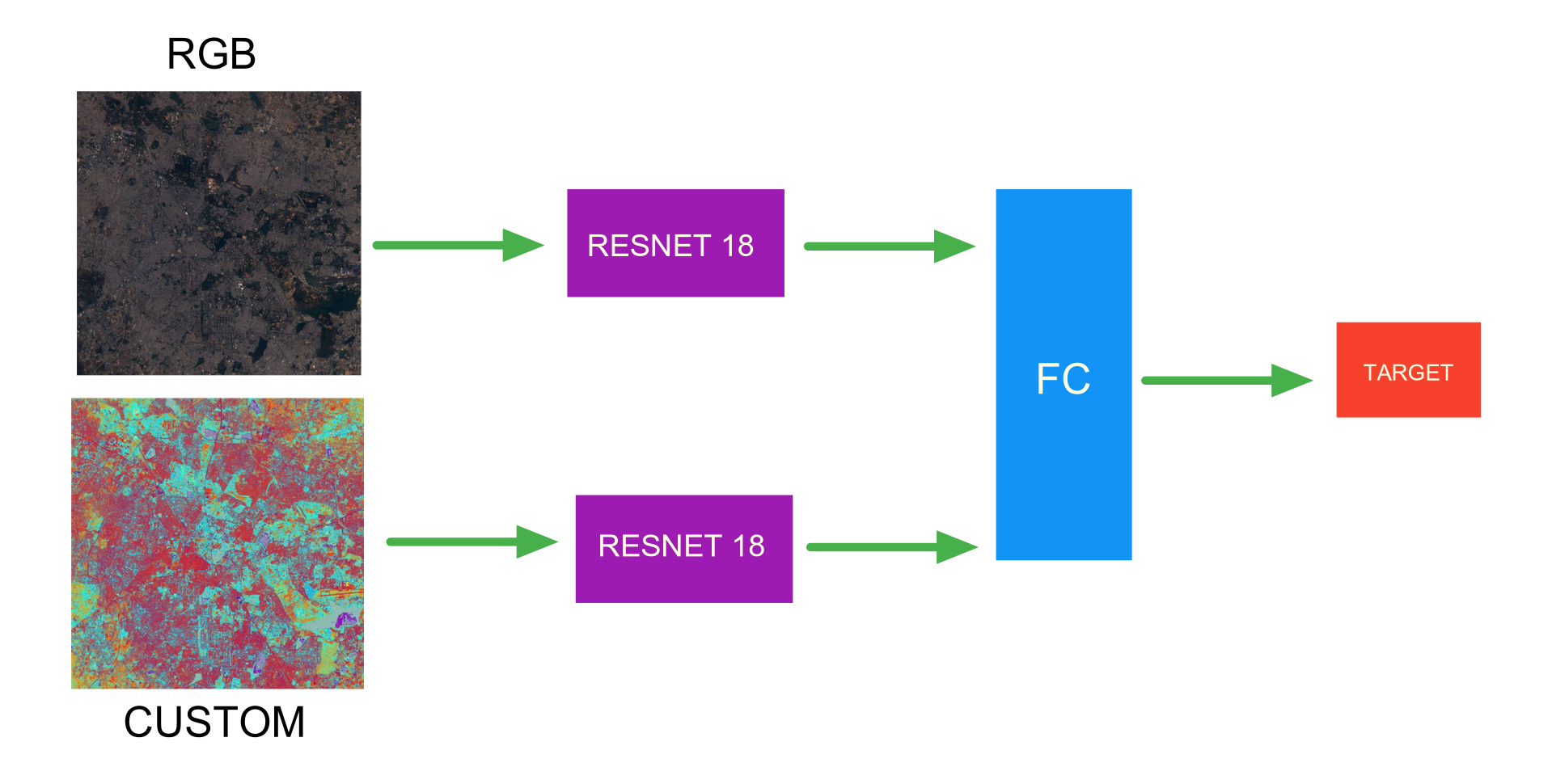The target is the AWI category which ranges from 0-4 (0 being very poor and 4 being very rich). It has been calculated by discretizing the AWI values. We decided to convert it into a classification problem by binning the AWI values as the AWI has a very high variance since we only have 640 or so images for modelling we decided that a classification approach would be better.

Now we can take a look at the forward method. This method essentially just reads each and every file from the provided image path list, creates a dictionary similar to the one that we created in the meta function and uploads the data in the form of arrays.

Let’s have a look at the code:

``````import rasterio
def forward(self, image_info):

"""
Returns a dictionary containing the files mentioned in
meta for every image path

:params image_info: list of dictionaries each containing
image path, target, names and awi

"""
ds = {}

# open the satellite image
image =  rasterio.open(image_info['image_path'])

# get the image inputs (RGB + CUSTOM)
_,_,_,rgb,comb = self.get_custom_bands(self, image)

# initialize the placeholders for the data and store them
ds['rgb-image'] = np.empty(1, object)
ds['rgb-image'] = rgb

ds['custom-image'] = np.empty(1, object)
ds['custom-image'] = comb

ds['awi'] = np.empty(1, dtype = 'float')
ds['awi'] = image_info['awi']

ds['name'] = np.empty(1, dtype = object)
ds['name'] = image_info['name']

ds['target'] = np.empty(1, dtype = 'float')
ds['target'] = image_info['target']

return ds``````

The satellite images are not stored in the system with our .jpg or .png formats, they have a special format called the GeoTiff format and the extension is .tif. To access such a file I have used rasterio, which is a python package for reading, visualizing and transforming satellite imagery.

The get_custom_band method takes care of calculating the NDVI, NDBI, NDWI indices, combines them and returns the RGB and the custom banded images. Even though understanding the code of this method is not our focus in this article, you can still have a look below:

``````@staticmethod
def get_custom_bands(self, image):

""" Returns custom 3 banded image by combining
NDVI/NDBI/NDWI
"""

# NDBI = (SWIR - NIR) / (SWIR + NIR)
nir = np.rollaxis(nir, 0, 3)

swir = np.rollaxis(swir, 0, 3)

# Do not display error when divided by zero
np.seterr(divide='ignore', invalid='ignore')

ndbi = np.zeros(nir.shape)
ndbi = (swir-nir) / (swir+nir)
ndbi = cv2.normalize(ndbi, None, alpha = 0, beta = 255, norm_type = cv2.NORM_MINMAX, dtype = cv2.CV_32F)
ndbi = ndbi[:,:,np.newaxis]

# RGB
rgb = cv2.normalize(rgb, None, alpha = 0, beta = 255, norm_type = cv2.NORM_MINMAX, dtype = cv2.CV_32F).transpose(1,2,0)
rgb = np.where(np.isnan(rgb), 0, rgb)
assert not np.any(np.isnan(rgb))

# NDVI = (NIR - Red) / (NIR + Red)
red = np.rollaxis(red, 0, 3)
ndvi = np.zeros(nir.shape)
ndvi = (nir-red)/(nir+red)
ndvi = cv2.normalize(ndvi, None, alpha = 0, beta = 255, norm_type = cv2.NORM_MINMAX, dtype = cv2.CV_32F)
ndvi = ndvi[:,:,np.newaxis]

# NDWI = (NIR - SWIR) / (NIR + SWIR)
ndwi = (nir-swir)/(nir+swir)
ndwi = cv2.normalize(ndwi, None, alpha = 0, beta = 255, norm_type = cv2.NORM_MINMAX, dtype = cv2.CV_32F)
ndwi = ndwi[:,:,np.newaxis]

# combined
comb = np.concatenate([ndbi, ndvi, ndwi], axis = -1)
comb = np.where(np.isnan(comb), 0, comb)
assert not np.any(np.isnan(comb))

return ndbi, ndvi, ndwi, rgb, comb``````

Another approach here could have been to upload all the bands i.e the Red, Green, Blue, SWIR and NIR bands instead of uploading the RGB and custom bands separately. While modelling, when we load the images we could have then calculated the required inputs using our ‘get_custom_bands’ method. This approach is more efficient according to me, but the only catch here is that we need 3 band images to visualize them on the app hence I stuck with the former approach.

Now we have created the class, the next step is to create the image_info dictionary, which contains everything that we want to upload, i.e. the image path from which we will get the rgb and custom images, the awi, the target and the name of the districts. We then create a dataset object using the dataset method provided by hub and the AWiGenerator class created above.

This load dataset function takes care of this:

``````def load_dataset(raster_path):
""" Creates the dataset object used to upload the data.

:param raster_path: The path where all the rasters are stored in the system """

get the train path and the test path
trainpath = os.path.join(rasterpath, 'Train') testpath = os.path.join(rasterpath, 'Test')

dataframe containing target
df = pd.readcsv('Data - Full/data-class.csv') trainimagepaths = os.listdir(trainpath) testimagepaths = os.listdir(test_path)

initialize image info list
imageinfolist = []

iterate over all images in train and test
tktrain = tqdm(trainimagepaths, total = len(trainimagepaths)) tktest = tqdm(testimagepaths, total = len(testimagepaths))

there are 543 training images
for image in tktrain: # image info dictionary for each image imageinfo = {}

# store the image path
image_info['image_path'] = os.path.join(train_path, image)

# getting the awi and name from raster name
awi = float(image.split('_')[:-4])
name = str(image.split('_'))
image_info['awi'] = awi
image_info['name'] = name

# get the target for the image
target = df['category'][(df['distname'] == name)
& (df['wealth_ind'] == awi)]
image_info['target'] = target

# check if the image is corrupted,
if it's not then append the image_info
try:
image = rasterio.open(image_info['image_path'])
image_info_list.append(image_info)

except Exception as e:
print(e)

We carry out the same process for the test data as well. Finally we get a list of dictionaries, image_info_list where the first 543 dictionaries are training and the rest correspond to testing set.

Once the list is ready, we simply call the generate command and return the dataset object.

``````ds = dataset.generate(AwiGenerator(), image_info_list)
return ds ``````

All the setup required to upload the data has been done! Now we just need to initialize our dataset object, name it and upload it using the store command.

``````path = 'Data - Full/'
# name of the data
ds.store('district-awi-2015-16-rgb-custom')``````

And we’re done! The data will get uploaded into your registered account with the name provided. Once the dataset has been uploaded we can actually see everything that has been uploaded using the visualizer app.

This is how the RGB images look like with their names.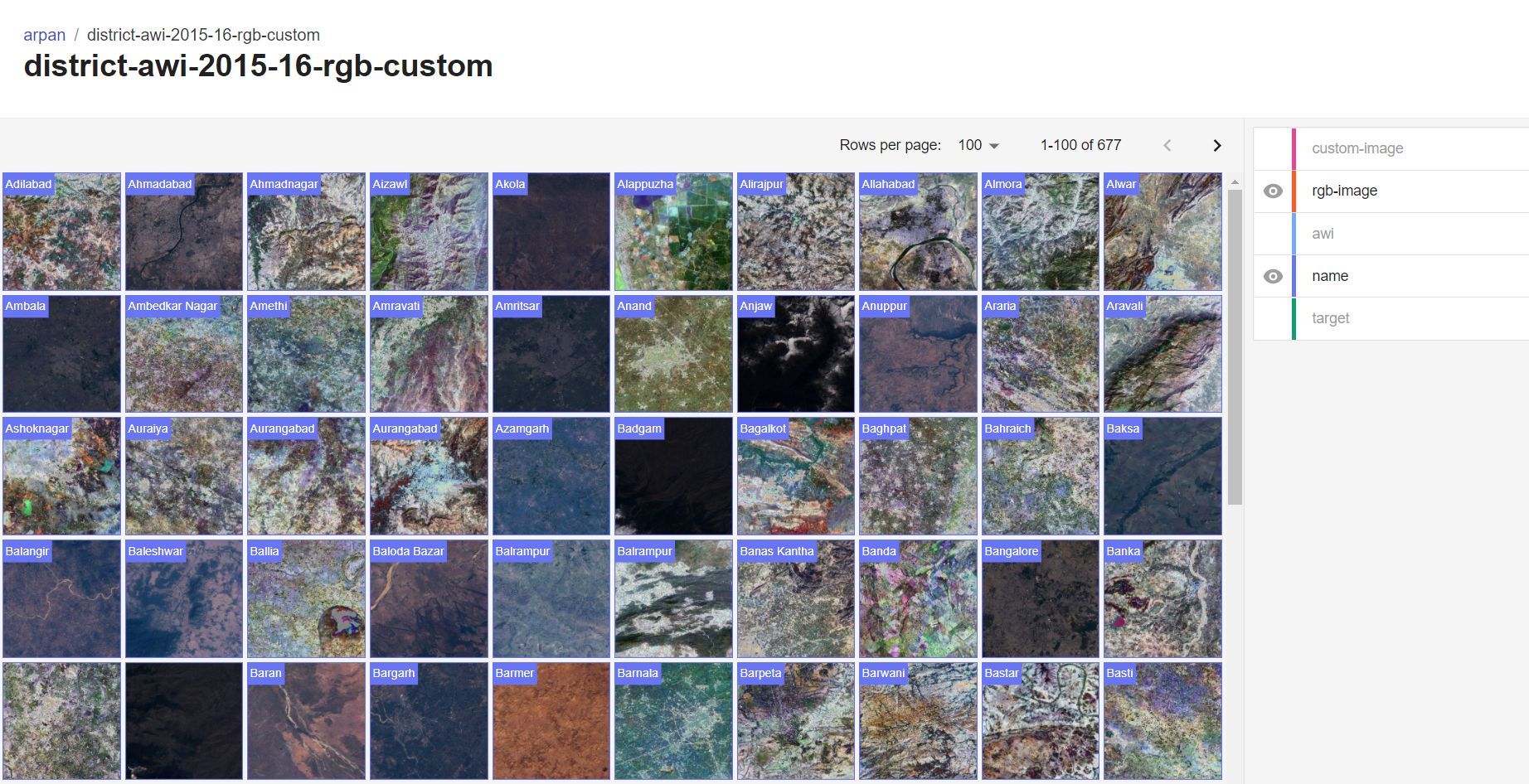We can see each one individually as well, here is Bangalore's rgb image and custom image with its target value.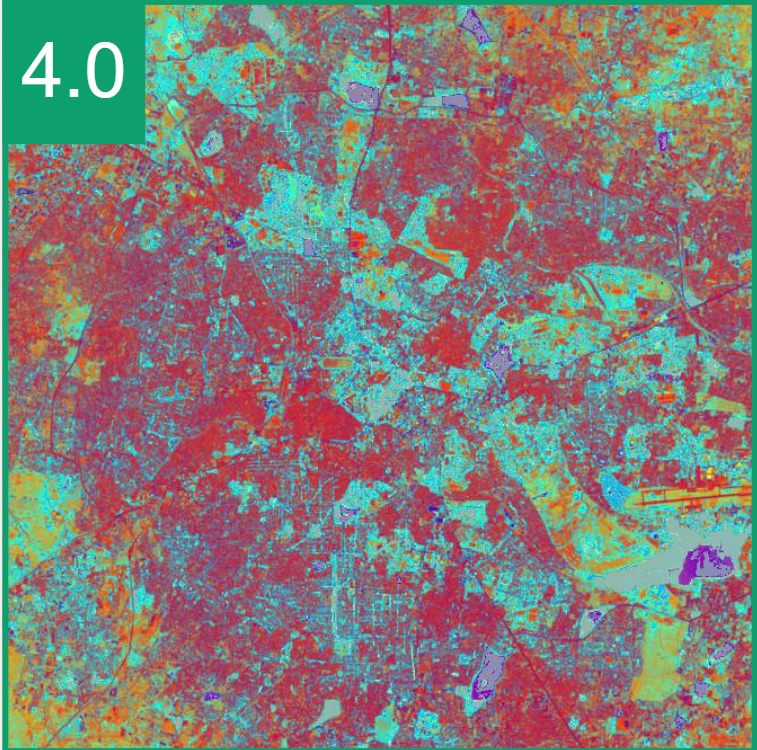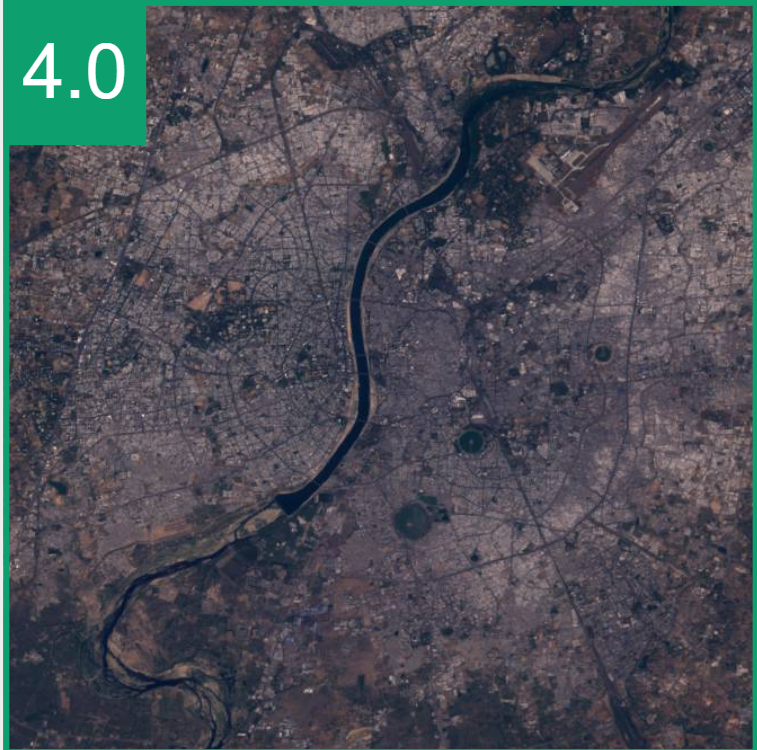You can play around with the entire dataset here. The tool helped me visualize any slice of the dataset with big satellite images I had almost instantly. This was handy for identifying buggy images and removing them. A bunch of popular datasets, such as mnist, fashion-mnist or CoCo pre-loaded in the visualization tool as well.

### Training Machine Learning models with hub

Now that we have uploaded the data, it can be accessed by anyone who has registered on the Activeloop platform using a single line of code.

``````import hub

Now ds is our dataset object, which can be used for doing all the processing on individual images as if they were stored on our own device, this is achieved using the Transform module provided by hub. For our use case, image augmentations are a necessary step since we have very less data and also the satellite images are not consistent, there might be cloud cover, the sun might be in a different angle when the image was taken by the satellite, the satellite itself could be in a different angle and so on. To make our model generalize better we have to use augmentations. The transform module makes it really easy to apply augmentations to our images. First we define our train time and test time augmentations using the albumentations package.

``````import albumentations
# imagenet stats
mean, std = [0.485, 0.456, 0.406], [0.229, 0.224, 0.225]

# training augmentations
aug = albumentations.Compose([
albumentations.Resize(512, 512, always_apply=True),
albumentations.Normalize(mean, std, always_apply = True),
albumentations.RandomBrightnessContrast(always_apply =   False),
albumentations.RandomRotate90(always_apply=False),
albumentations.HorizontalFlip(),
albumentations.VerticalFlip()])
# testing augmentations
aug_test = albumentations.Compose([
albumentations.Resize(512, 512, always_apply=True),
albumentations.Normalize(mean, std, always_apply = True)])``````

Now we apply the training augmentations to the training images one by one and then similarly, test time augmentations to the testing images.

For that, we will create a transformer class like so:

``````class TrainTransformer(Transform):
def meta(self):
return {
'rgb-image': {"shape": (1,), "dtype": "object", 'dtag': 'image'},
'custom-image': {"shape": (1,), "dtype": "object", 'dtag': 'image'},
'target': {'shape': (1, ), 'dtype': 'float', 'dtag': 'text'}
}

def forward(self, item):
ds = {}

# load rgb and apply augmentations
ds['rgb-image'] = np.empty(1, object)
rgb = item['rgb-image']
ds['rgb-image'] = aug(image = rgb)['image'].transpose(2,0,1)

# load custom and apply augmentations
ds['custom-image'] = np.empty(1, object)
custom = item['custom-image']
ds['custom-image'] = aug(image = custom)['image'].transpose(2,0,1)

ds['target'] = np.empty(1, dtype = 'float')
ds['target'] = item['target']
return ds``````

This is quite similar to the generator class we created for uploading images. We have a meta method which defines all files that we want to use for training (we are not using name and awi as they are not required) and we have a forward method which applies augmentations to our images one by one and returns the data in the form of a dictionary.

We follow the exact same steps to create a TestTransformer.

Now we have to initialize our training and testing dataset and convert them to either PyTorch or TensorFlow format. I have used Fast AI 2.0 which is built on top of PyTorch for training models and hence I have converted the dataset into PyTorch format. FastAI also provides some advanced functionalities like learning rater finder, gradual unfreezing, discriminative learning rates etc. These would normally take a lot more code to implement in PyTorch. FastAI + Hub, running machine learning experiments has never been easier!

``````# --------------------------------------------------------
num_train_samples = 543
train_ds = dataset.generate(TrainTransformer(), ds[0:num_train_samples])
test_ds = dataset.generate(TestTransformer(), ds[num_train_samples:])
# --------------------------------------------------------

# convert to pytorch
train_ds = train_ds.to_pytorch(lambda x:((x['rgb-image'], x['custom-image']), x['target']))
test_ds = test_ds.to_pytorch(lambda x:((x['rgb-image'], x['custom-image']), x['target']))

When we uploaded the dataset we had seen that the first 543 samples were training data points hence the variable num_train_samples takes on that value. Once the dataset has been created we use our standard PyTorch data loader wrapper to create train and test loaders. Now we are ready to create our model. First, we create our model architecture which uses 2 resnet 18 feature extractors (body) and then a fully connected layer (head).

class AWIModel(nn.Module): def init(self,arch, ps = 0.5, input_fts = 1024): super(AWIModel, self).init()

``````# resnet 18 feature extractors
self.body1 = create_body(arch, pretrained=True)
self.body2 = create_body(arch, pretrained=True)
# fully connected layers

def forward(self, X):
x1, x2 = X
x1 = self.body1(x1)
x2 = self.body2(x2)
x = torch.cat([x1,x2], dim = 1)

return x``````

Next we define our loss function, fastai’s Dataloaders object and create our learn object.

``````dls = DataLoaders(train_loader, test_loader)
model = AWIModel(arch = models.resnet18, ps = 0.2)
learn = Learner(dls = dls, model = model, loss_func = loss_fnc, opt_func = Adam, metrics = [accuracy], cbs = CudaCallback(device = 'cuda'))``````

As we have everything, we can start training our model by selecting an appropriate learning rate.

``learn.fit_one_cycle(6, lr_max =  5e-4)``

### Conclusion: the fastest solution for efficient machine learning and scalable data pipelines

We went through an entire machine learning pipeline that can be created using the hub package. The data is stored in a central location, to be easily used by a team of machine learning engineers for loading the data and conducting experiments with it. Notably, the data can be accessed and modified as fast as if it were on premise. Datasets that would otherwise take 30-40 hours to download and prepare take 2 minutes to access with hub. Once uploaded, all imagery datasets can be visualized to allow for some exploration and debugging. All this saved my team about 2 weeks worth of time, which is huge for a project with an allocated time of 8 weeks.

In all, Activeloop’s open-source solution is built for distributed teams that need to get results fast at the lowest cost. Satellite imagery is just the tip of the iceberg. The open-source stack has many more functionalities for scalable data pipelines and easier dataset management. For instance, with the new v1.0 update, storing the data, as well as dataset preprocessing are looking to get even easier. Check out their website and documentation to learn more, and join Activeloop’s Slack community to ask the team more questions!

Unifying and abstracting away infrastructure for easier and highly efficient machine and deep learning.